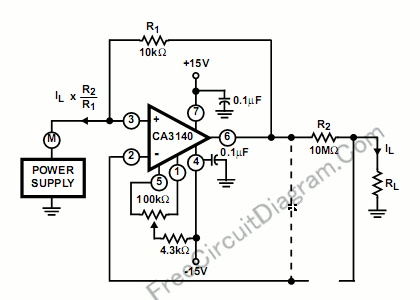# Current Amplifier Using CA3140 Op-AmpCurrent amplifier application need very low input bias current, and CA3140 is one of suitable op-amps for this purpose. The circuit’s schematic diagram is shown in the figure below.In the circuit, low current is supplied at the input potential as the power supply to load resistor RL. When the load current is monitored by the power supply meter M, this load current is increased by the multiplication factor R2/R1. With values shown, if the load current is 100nA,  the load current presented to the supply will be 100µA, a much easier current to measure using many low cost meters.

Remember that the input and output voltages are transferred at the same potential and only the output current is amplified by the scale factor. The dotted components show a decoupling capacitor fto prevent potentioal oscillation at high output load capacitance.  The point is, the required high frequency feedback is provided by the capacitor with the dotted series resistor providing load decoupling. [Circuit’s schematic diagram source: Intersil’s CA3140 Application Notes]